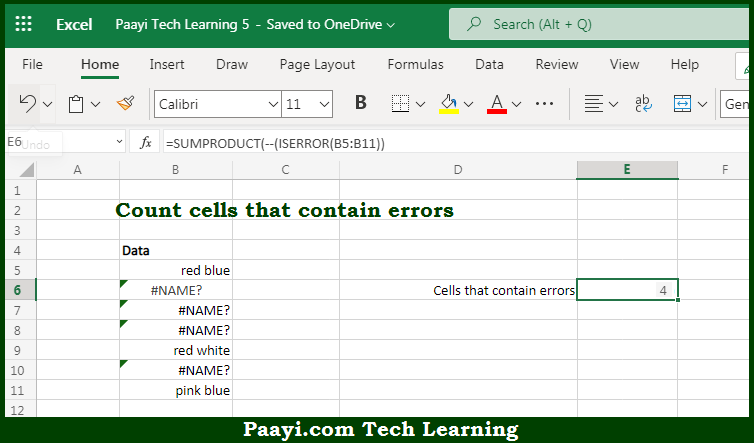# Learn How to Count Cells that Contain Errors in Microsoft Excel

Written by | 0 Comments | 577 Views

In this article, you will learn how to COUNT various things in Microsoft Excel using a single or combination of functions and its purpose in Microsoft Excel. You will also get to know how to count cells that contain errors and see the generic formula.

Count Cells that Contain Errors in Microsoft Excel

The main purpose of this formula is to count cells that contain errors. Here we will learn how to count cells having any kind of error in Microsoft Excel.  That implies, with the help of a formula based on the  SUMPRODUCT and ISERROR function you can able to count the number of cells that contain having some kind of error. So, with the help of this formula, you can able to count cells containing any kind of error.

General Formula to COUNT Cells that Contain Errors

=SUMPRODUCT(--ISERROR(range))

The Explanation for the COUNT Cells that Contain ErrorsSo we know that with the help of the given formula above you can able to count cells that contain errors. Here we will learn how to count cells having any kind of error in Microsoft Excel. As we know that, when you use the SUMPRODUCT function it accepts one or more arrays, multiplies the arrays together, and returns the "sum of products" as a final result. It should be noted that if only one array is supplied, the SUMPRODUCT function will simply return the sum of items in the array. So, with the help of this formula, you can able to count cells that contain errors.